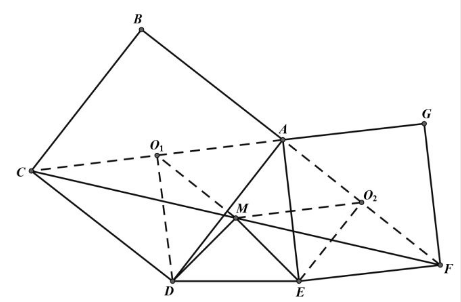【答案】

$A E F G$ 的中心, 则
\begin{aligned} & O_1 M / / A F, O_2 M / / A C \\ & O_1 M=A O_2=O_2 E, \\ & O_2 M=A O_1=O_1 D, \\ & \angle D O_1 M=90^{\circ}-\angle A O_1 M=90^{\circ}- \\ & \angle A O_2 M=\angle M O_2 E, \\ & \therefore \triangle D O_1 M \cong \triangle M O_2 E, M D=E M \\ & \text { 又 } O_1 M \perp O_2 E, O_2 M \perp O_1 D, \therefore M D \\ & \perp E M \end{aligned}